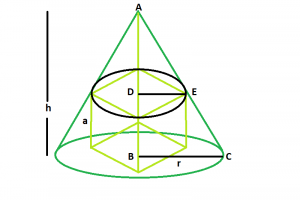# Largest cube that can be inscribed within a right circular cone

Given a right circular cone of radius r and perpendicular height h. We have to find the side length of the biggest cube that can be inscribed within it.

Examples:

```Input : h = 5, r = 6
Output : 3.14613

Input : h = 8, r = 12
Output : 5.43698
```## Recommended: Please try your approach on {IDE} first, before moving on to the solution.

Approach:
Let, side of the cube = a.

From the diagram, we can clearly understand using the properties of triangles: BC/AB = DE/AD.

Therefore,

```r/h = (a/√2)/(h-a)

or, a = h*r√2/(h+√2*r)
```

Below is the implementation of the above approach:

## C++

 `// C++ Program to find the biggest cube ` `// inscribed within a right circular cone ` `#include ` `using` `namespace` `std; ` ` `  `// Function to find the side of the cube ` `float` `cubeSide(``float` `h, ``float` `r) ` `{ ` `    ``// height and radius cannot be negative ` `    ``if` `(h < 0 && r < 0) ` `        ``return` `-1; ` ` `  `    ``// side of the cube ` `    ``float` `a = (h * r * ``sqrt``(2)) / (h + ``sqrt``(2) * r); ` ` `  `    ``return` `a; ` `} ` ` `  `// Driver code ` `int` `main() ` `{ ` `    ``float` `h = 5, r = 6; ` ` `  `    ``cout << cubeSide(h, r) << endl; ` ` `  `    ``return` `0; ` `} `

## Java

 `// Java Program to find the the biggest cube  ` `// which can be inscribed within a right circular cone ` ` `  `import` `java.io.*;  ` ` `  `class` `GFG {  ` ` `  ` `  `// Function to find the side of the cube ` ` `  `static` `float` `cube(``float` `h, ``float` `r)  ` `{  ` ` `  `    ``// hegiht and radius cannot be negative  ` `    ``if` `(h < ``0` `&& r < ``0``)  ` `        ``return` `-``1``;  ` ` `  ` ``// side of the cube  ` `    ``float` `a = (h * r * (``float``)Math.sqrt(``2``)) / (h + (``float``)Math.sqrt(``2``) * r);  ` `   `  `    ``return` `a;  ` `}  ` ` `  `// Driver code  ` `   `  `    ``public` `static` `void` `main (String[] args) {  ` `          ``float` `h = ``5``, r = ``6``; ` `    ``System.out.println( cube(h, r));  ` `    ``}  ` `}  ` ` `  `// this article is contributed by Ishwar Gupta `

## Python 3

 `# Python3 Program to find the biggest cube ` `# inscribed within a right circular cone ` `import` `math ` ` `  `# Function to find the side of the cube ` `def` `cubeSide(h, r): ` ` `  `    ``# height and radius cannot  ` `    ``# be negative ` `    ``if` `(h < ``0` `and` `r < ``0``): ` `        ``return` `-``1` ` `  `    ``# side of the cube ` `    ``a ``=` `((h ``*` `r ``*` `math.sqrt(``2``)) ``/`  `         ``(h ``+` `math.sqrt(``2``) ``*` `r)) ` ` `  `    ``return` `a ` ` `  `# Driver code ` `h ``=` `5``; r ``=` `6``; ` ` `  `print``(cubeSide(h, r), ``"\n"``) ` ` `  `# This code is contributed  ` `# by Akanksha Rai `

## C#

 `// C# Program to find the the  ` `// biggest cube which can be  ` `// inscribed within a right  ` `// circular cone ` `using` `System; ` ` `  `class` `GFG  ` `{  ` ` `  `// Function to find the side  ` `// of the cube ` `static` `float` `cube(``float` `h, ``float` `r)  ` `{  ` ` `  `// hegiht and radius cannot be negative  ` `if` `(h < 0 && r < 0)  ` `    ``return` `-1;  ` ` `  `// side of the cube  ` `float` `a = (h * r * (``float``)Math.Sqrt(2)) /  ` `          ``(h + (``float``)Math.Sqrt(2) * r);  ` ` `  `    ``return` `a;  ` `}  ` ` `  `// Driver code  ` `public` `static` `void` `Main ()  ` `{  ` `    ``float` `h = 5, r = 6; ` `    ``Console.Write( cube(h, r));  ` `}  ` `} ` ` `  `// This code is contributed  ` `// by 29AjayKumar `

## PHP

 ` `

Output:

```3.14613
```

Don’t stop now and take your learning to the next level. Learn all the important concepts of Data Structures and Algorithms with the help of the most trusted course: DSA Self Paced. Become industry ready at a student-friendly price.

My Personal Notes arrow_drop_upCheck out this Author's contributed articles.

If you like GeeksforGeeks and would like to contribute, you can also write an article using contribute.geeksforgeeks.org or mail your article to contribute@geeksforgeeks.org. See your article appearing on the GeeksforGeeks main page and help other Geeks.

Please Improve this article if you find anything incorrect by clicking on the "Improve Article" button below.

Article Tags :
Practice Tags :

Be the First to upvote.

Please write to us at contribute@geeksforgeeks.org to report any issue with the above content.